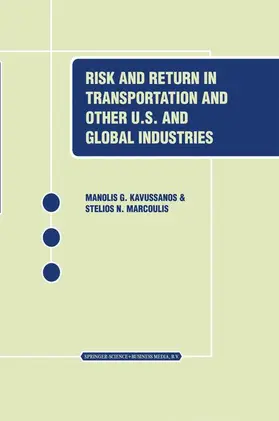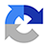# Marcoulis / KavussanosRisk and Return in Transportation and Other US and Global Industries

2001, 174 Seiten, Gebunden, HC runder Rücken kaschiert, Format (B × H): 160 mm x 241 mm, Gewicht: 1000 g
ISBN: 978-0-7923-7356-8
Verlag: Springer US

## Marcoulis / KavussanosRisk and Return in Transportation and Other US and Global Industries

The type of research methodologies used in analysing stock returns in the book is outlined in this technical chapter. The chapter begins with definitions of useful summary statistics, such as the mean, standard deviation, coefficient of variation, and their interpretation. Tests statistics for inferences on population means, proportions and differences of means, among others, are also presented. A summary of single equation regression techniques and the way these are applied through estimation and inference are outlined, focusing on the interpretation of standard output and diagnostic tests. The single equation methodology is then extended to multiple equation systems; the methods of Multivariate Least Square (MLSQ) of Seemingly Unrelated Regression Equations (SURE) are Regressions as well as those considered. Time series, ARlMA, models form also part of the discussion. This is a chapter, which is aimed at readers who are interested in understanding the technical background used in deriving results later in the book. 2.2. Summary Statistics for a set of Data A set of numbers which are generated by agents' actions in the market, and which can take different values if the agents were to repeat their actions, are known as random variables. For example, prices of shares in the stock exchange are determined at each point in time from the collective actions of agents operating in the market through their demand and supply decisions. The price of a share is thus a random variable, and so is the return of the share.

Research

## Weitere Infos & Material

List of Figures. List of Tables. List of Abbreviations. List of Symbols. 1. Book Overview. 2. Statistical Methodology. 3. Data Definitions, Properties, Transformations. 4. Results from the CAPM - Capital Asset Pricing Model. 5. Microeconomic (Company Specific) Factors As Determinants of Equity Returns. 6. Macroeconomic (Economy Wide) Factors As Determinants of Equity Returns. 7. Microeconomic and Macroeconomic Factors - A Unified Approach. 8. Macroeconomic Factors and International Industry Returns. 9. Conclusion. List of Figures.

## Ihre Fragen, Wünsche oder Anmerkungen

Ihre Nachricht*
Wie möchten Sie kontaktiert werden?
Anrede*
Titel
Vorname*
Nachname*